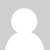### Matlab

Hey. Does anyone have an idea how to transform the Emonlib library code to Matlab?

The idea is to send via serial port the values that the arduino is reading in the analog pins, the instantaneous values and then do all the maths, calibration and processing in the Matlab, I am asking this because this would increase the velocity of data acquisition (less calculations in the arduino), but I am having some problems in adapting the arduino code to Matlab.

Is there any matlab expert that could help me with this.

I also thing this would be good to others, that is why I am sharing my idea and problem.

Thank you

### Re: Matlab

Hello, I'm afraid the speed of the serial coms is much slower than the sample rate and calculation, it will be the serial coms that will be the bottleneck rather than the math calculations.### Re: Matlab

I suppose you could gather a few thousand points then transmit all at once. Unfortunately I'm not a MATLAB expert, but their website seems to document their Serial Port functionality quite well. Try following the examples and modify accordingly.

http://www.mathworks.com/help/techdoc/matlab_external/f92576.html### Re: Matlab

But I have a question, how did you obtain the data to plot the graphics on this page http://openenergymonitor.org/emon/buildingblocks/ac-power-arduino-maths .

When I try to put in the serial the instantaneous values of voltage and current I just obtain zeros.